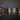# Mini-Symposium: Advances in K-Theory, Symmetry, and Periodicity

This Mini-Symposium is part of the DMV Annual Meeting 2022 to be held on September 12-16 in Berlin, Germany. The Mini-symposium will take place on September 16th at 10:30-12:30 and 15:30-17:30.

## Speakers

• Jack Morgan Davies, Utrecht University
• Eva Höning, Radboud University
• Mikala Ørsnes Jansen, University of Copenhagen
• J.D. Quigley, MPI Bonn/University of Oregon

## Description

Interactions between the fields of algebraic K-theory, equivariant homotopy theory, and chromatic homotopy theory, have led to exciting new results with applications to number theory and geometric topology. The goal of this Minisymposium is to highlight recent advances in Hochschild homology, algebraic K-theory, equivariant homotopy theory, and chromatic homotopy theory that combine tools from several of these fields, as well as bring together researchers from these different areas to promote future interactions between these fields.

## Abstracts:

Jack Morgan Davies, Utrecht University

Stable operations in elliptic cohomology

Many famous results in topology can be proven using operations on various cohomology theories. In this talk, we will discuss two families of stable operations on elliptic cohomology and topological modular forms. These include stable Adams operations, analogous to those on topological K-theory, and stable Hecke operators, inspired by operations in number theory. We will see in some small examples how homotopy theoretic techniques can be used to perform number theoretic calculations.

Eva Höning, Radboud University

Algebraic K-theory of the second truncated Brown-Peterson spectrum

This is a report on joint work with G. Angelini-Knoll, Ch. Ausoni, D. Culver and J. Rognes. I will explain how to prove a strong form of the redshift conjecture for $BP \langle 2 \rangle$ by explicitly computing the mod $(p, v_1, v_2)$ homotopy of its topological cyclic homology.

Mikala Ørsnes Jansen, University of Copenhagen

Unstable algebraic K-theory

Let R by a ring. The term unstable algebraic K-theory will refer to any (family of) anima K(R,n) built entirely out of linear algebra internal to R^n through which the canonical maps BGL_n(R) –> K(R) factorise. A classical example is Quillen's plus-construction BGL_n(R)^+. Ideally, we want a model for unstable algebraic K-theory to be closer to K(R) than BGL_n(R) is in terms of its nature and properties; for example the fundamental group of the plus-construction is closer to K_1(R) than GL_n(R) is. The term unstable algebraic K-theory was used in the 1970's by Dennis and Stein in a survey of the functor K_2, and classically unstable algebraic K-theory has been used to derive many important computational results about ''stable'' algebraic K-theory. We introduce a new model for unstable algebraic K-theory inspired by a detailed study of the so-called reductive Borel--Serre compactification of locally symmetric spaces. In this talk I will mention the main results and calculations of this work and also attempt to shed light on the geometric origins of the model, as this is an important and very interesting aspect of the story. This is joint work with Dustin Clausen.

J.D. Quigley, MPI Bonn/University of Oregon

Bredon homological stability

Homological stability is a well-studied phenomenon with applications to stable homotopy theory and algebraic K-theory. In this talk, I will introduce Bredon homological stability, an equivariant refinement of homological stability, and discuss some examples. This is joint work with Eva Belmont.